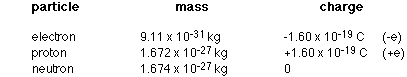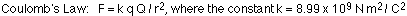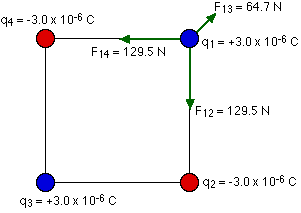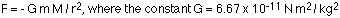## Electric charge and Coulomb's law

7-6-99

### Charge

• there are two kinds of charge, positive and negative
• like charges repel, unlike charges attract
• positive charge comes from having more protons than electrons; negative charge comes from having more electrons than protons
• charge is quantized, meaning that charge comes in integer multiples of the elementary charge e
• charge is conserved

Probably everyone is familiar with the first three concepts, but what does it mean for charge to be quantized? Charge comes in multiples of an indivisible unit of charge, represented by the letter e. In other words, charge comes in multiples of the charge on the electron or the proton. These things have the same size charge, but the sign is different. A proton has a charge of +e, while an electron has a charge of -e.

Electrons and protons are not the only things that carry charge. Other particles (positrons, for example) also carry charge in multiples of the electronic charge. Those are not going to be discussed, for the most part, in this course, however.

Putting "charge is quantized" in terms of an equation, we say:

q = n e

q is the symbol used to represent charge, while n is a positive or negative integer, and e is the electronic charge, 1.60 x 10-19 Coulombs.

### The Law of Conservation of Charge

The Law of conservation of charge states that the net charge of an isolated system remains constant.

If a system starts out with an equal number of positive and negative charges, thereąs nothing we can do to create an excess of one kind of charge in that system unless we bring in charge from outside the system (or remove some charge from the system). Likewise, if something starts out with a certain net charge, say +100 e, it will always have +100 e unless it is allowed to interact with something external to it.

Charge can be created and destroyed, but only in positive-negative pairs.

Table of elementary particle masses and charges:### Electrostatic charging

Forces between two electrically-charged objects can be extremely large. Most things are electrically neutral; they have equal amounts of positive and negative charge. If this wasnąt the case, the world we live in would be a much stranger place. We also have a lot of control over how things get charged. This is because we can choose the appropriate material to use in a given situation.

Metals are good conductors of electric charge, while plastics, wood, and rubber are not. Theyąre called insulators. Charge does not flow nearly as easily through insulators as it does through conductors, which is why wires you plug into a wall socket are covered with a protective rubber coating. Charge flows along the wire, but not through the coating to you.

Materials are divided into three categories, depending on how easily they will allow charge (i.e., electrons) to flow along them. These are:

• conductors - metals, for example
• semi-conductors - silicon is a good example
• insulators - rubber, wood, plastic for example

Most materials are either conductors or insulators. The difference between them is that in conductors, the outermost electrons in the atoms are so loosely bound to their atoms that theyąre free to travel around. In insulators, on the other hand, the electrons are much more tightly bound to the atoms, and are not free to flow. Semi-conductors are a very useful intermediate class, not as conductive as metals but considerably more conductive than insulators. By adding certain impurities to semi-conductors in the appropriate concentrations the conductivity can be well-controlled.

There are three ways that objects can be given a net charge. These are:

1. Charging by friction - this is useful for charging insulators. If you rub one material with another (say, a plastic ruler with a piece of paper towel), electrons have a tendency to be transferred from one material to the other. For example, rubbing glass with silk or saran wrap generally leaves the glass with a positive charge; rubbing PVC rod with fur generally gives the rod a negative charge.
2. Charging by conduction - useful for charging metals and other conductors. If a charged object touches a conductor, some charge will be transferred between the object and the conductor, charging the conductor with the same sign as the charge on the object.
3. Charging by induction - also useful for charging metals and other conductors. Again, a charged object is used, but this time it is only brought close to the conductor, and does not touch it. If the conductor is connected to ground (ground is basically anything neutral that can give up electrons to, or take electrons from, an object), electrons will either flow on to it or away from it. When the ground connection is removed , the conductor will have a charge opposite in sign to that of the charged object.

An example of induction using a negatively charged object and an initially-uncharged conductor (for example, a metal ball on a plastic handle).

(1) bring the negatively-charged object close to, but not touching, the conductor. Electrons on the conductor will be repelled from the area nearest the charged object.

(2) connect the conductor to ground. The electrons on the conductor want to get as far away from the negatively-charged object as possible, so some of them flow to ground.

(3) remove the ground connection. This leaves the conductor with a deficit of electrons.

(4) remove the charged object. The conductor is now positively charged.

A practical application involving the transfer of charge is in how laser printers and photocopiers work.

### Why is static electricity more apparent in winter?

You notice static electricity much more in winter (with clothes in a dryer, or taking a sweater off, or getting a shock when you touch something after walking on carpet) than in summer because the air is much drier in winter than summer. Dry air is a relatively good electrical insulator, so if something is charged the charge tends to stay. In more humid conditions, such as you find on a typical summer day, water molecules, which are polarized, can quickly remove charge from a charged object.

### Try this at home

See if you can charge something at home using friction. I got good results by rubbing a Bic pen with a piece of paper towel. To test the charge, you can use a narrow stream of water from a faucet; if the object attracts the stream when it's brought close, you know it's charged. All you need to do is to find something to rub - try anything made out of hard plastic or rubber. You also need to find something to rub the object with - potential candidates are things like paper towel, wool, silk, and saran wrap or other plastic.

### Coulomb's law

The force exerted by one charge q on another charge Q is given by Coulomb's law:r is the distance between the charges.

Remember that force is a vector, so when more than one charge exerts a force on another charge, the net force on that charge is the vector sum of the individual forces. Remember, too, that charges of the same sign exert repulsive forces on one another, while charges of opposite sign attract.

### An example

Four charges are arranged in a square with sides of length 2.5 cm. The two charges in the top right and bottom left corners are +3.0 x 10-6 C. The charges in the other two corners are -3.0 x 10-6 C. What is the net force exerted on the charge in the top right corner by the other three charges?To solve any problem like this, the simplest thing to do is to draw a good diagram showing the forces acting on the charge. You should also let your diagram handle your signs for you. Force is a vector, and any time you have a minus sign associated with a vector all it does is tell you about the direction of the vector. If you have the arrows giving you the direction on your diagram, you can just drop any signs that come out of the equation for Coulomb's law.

Consider the forces exerted on the charge in the top right by the other three:You have to be very careful to add these forces as vectors to get the net force. In this problem we can take advantage of the symmetry, and combine the forces from charges 2 and 4 into a force along the diagonal (opposite to the force from charge 3) of magnitude 183.1 N. When this is combined with the 64.7 N force in the opposite direction, the result is a net force of 118 N pointing along the diagonal of the square.The symmetry here makes things a little easier. If it wasn't so symmetric, all you'd have to do is split the vectors up in to x and y components, add them to find the x and y components of the net force, and then calculate the magnitude and direction of the net force from the components. Example 16-4 in the textbook shows this process.

### The parallel between gravity and electrostatics

An electric field describes how an electric charge affects the region around it. It's a powerful concept, because it allows you to determine ahead of time how a charge will be affected if it is brought into the region. Many people have trouble with the concept of a field, though, because it's something that's hard to get a real feel for. The fact is, though, that you're already familiar with a field. We've talked about gravity, and we've even used a gravitational field; we just didn't call it a field.

When talking about gravity, we got into the (probably bad) habit of calling g "the acceleration due to gravity". It's more accurate to call g the gravitational field produced by the Earth at the surface of the Earth. If you understand gravity you can understand electric forces and fields because the equations that govern both have the same form.

The gravitational force between two masses (m and M) separated by a distance r is given by Newton's law of universal gravitation:A similar equation applies to the force between two charges (q and Q) separated by a distance r:The force equations are similar, so the behavior of interacting masses is similar to that of interacting charges, and similar analysis methods can be used. The main difference is that gravitational forces are always attractive, while electrostatic forces can be attractive or repulsive. The charge (q or Q) plays the same role in the electrostatic case that the mass (m or M) plays in the case of the gravity.

A good example of a question involving two interacting masses is a projectile motion problem, where there is one mass m, the projectile, interacting with a much larger mass M, the Earth. If we throw the projectile (at some random launch angle) off a 40-meter-high cliff, the force on the projectile is given by:

F = mg

This is the same equation as the more complicated equation above, with G, M, and the radius of the Earth, squared, incorporated into g, the gravitational field.

So, you've seen a field before, in the form of g. Electric fields operate in a similar way. An equivalent electrostatics problem is to launch a charge q (again, at some random angle) into a uniform electric field E, as we did for m in the Earth's gravitational field g. The force on the charge is given by F = qE, the same way the force on the mass m is given by F = mg.

We can extend the parallel between gravity and electrostatics to energy, but we'll deal with that later. The bottom line is that if you can do projectile motion questions using gravity, you should be able to do them using electrostatics. In some cases, youąll need to apply both; in other cases one force will be so much larger than the other that you can ignore one (generally if you can ignore one, it'll be the gravitational force).